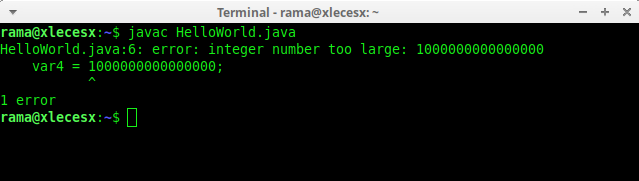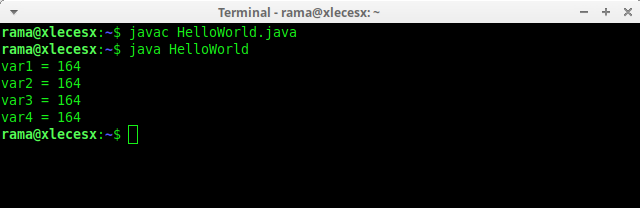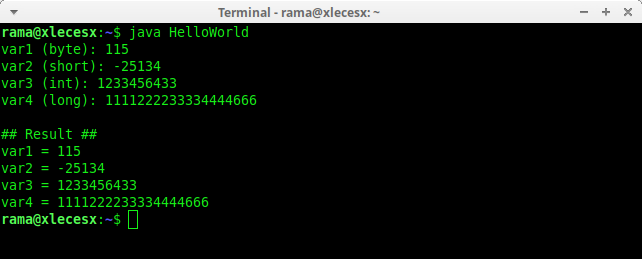# Integer Data Type Java Language With ExampleToday, we will discuss the understanding and how to use Integer data types in java programming language.

### Understanding the Integer Type of Java Language

Integer data types are data types used to hold positive or negative integers, such as: 4, 30, and -1234. There are 4 sub-types of integers which are distinguished based on the range of numbers in the Java language:

• byte
• short
• int
• long

The following table summarizes the range of each integer data type in Java:
Data Type Storage Memory Size Range
byte 1 byte -128 to 127
short 2 byte -32,768 to 32,767
int 4 byte -2,147,483,648 to 2,147,483,647
long 8 byte< -9,223,372,036,854,775,808 to 9,223,372,036,854,775,807

Memory Storage Size is the amount of memory needed to store these numbers. The greater the range, the more memory space is needed.

The Java language also does not recognize unsigned integers like in C or C ++ languages. Unsigned is a way to sacrifice negative values to increase the range of positive numbers. That is, in Java integer data types will always have negative and positive numbers.

Example Code Program Data Type Java Integer Language

In the following program code below, I use the 4 integer data types:
``````
class HelloWorld {
public static void main(String args[]){

byte  var1;
short var2;
int   var3;
long  var4;

var1 = 120;
var2 = 32000;
var3 = 1000000000;
var4 = 1000000000000000L;

System.out.println("var1 = "+var1);
System.out.println("var2 = "+var2);
System.out.println("var3 = "+var3);
System.out.println("var4 = "+var4);
}
}
``````

In rows 4 - 7, there are four variable declaration processes with different integer data types, namely byte data types for var1, short for var2, int for var3 and long for var4.

These four variables are then assigned with the values in lines 9-12, and then displayed with the System.out.println() command in lines 14-17. The following results:
``````
var1 = 120
var2 = 32000
var3 = 1000000000
var4 = 1000000000000000
``````

Var4 with long data type, the filling process must add the character "L" at the end, i.e. var4 = 1000000000000000L.

This additional "L" character is required because by default integers in Java are considered int. Long data types without the addition of "L" will produce an error:
``````
class HelloWorld {
public static void main(String args[]){

long  var4;

var4 = 1000000000000000;

System.out.println("var4 = "+var4);
}
}
``````

Error Message:### Writing Binary, Octal and Hexadecimal Numbers in Java

For certain purposes, sometimes we need to process numbers in binary, octal, and hexadecimal number systems. This can also be accommodated by Java language integer data types.

Binary is a designation for a number system consisting of 2 digits, namely 0 and 1. To input this number, add the prefix "0b" before writing the number, such as 0b10100100.

Octal is a number system consisting of 8 digit numbers, i.e., 1, 2, 3, 4, 5, 6, and 7. To make octal numbers in Java, add the prefix "0" before writing numbers, such as 0244.

Hexadecimal is a number system consisting of 16 digit numbers, i.e. 0-9 and the letter A-F. To make hex numbers in Java, add the prefix "0x" before writing numbers, like 0xA4.

Also, the everyday number system, which is 10 digits 0-9, is called a decimal number.

Here is an example program code for writing binary, octal, decimal and hexadecimal numbers in Java:
``````
class HelloWorld {
public static void main(String args[]){

int var1, var2, var3, var4;

var1 = 0b10100100;    // binary literal
var2 = 0244;          // octal literal
var3 = 164;           // decimal literal
var4 = 0xA4;          // hexadecimal literal

System.out.println("var1 = "+var1);
System.out.println("var2 = "+var2);
System.out.println("var3 = "+var3);
System.out.println("var4 = "+var4);
}
}
``````

Program code results:In the program code above, I created 4 variables: var1, var2, var3, and var4 which are all set as int data types. Then in lines 6-9 these four variables are filled with numbers 0b10100100, 0244, 164, and 0xA4.

When the program results are displayed, all variables will be converted into decimal numbers. This means, binary numbers 10100100, octal 244, and hex A4 are all converted to 164 in decimal numbers.

Note: be careful with writing numbers with the leading zero, like 0123. If you write like that, then Java thinks it's a number 123 in octal format, and not the same as a decimal number 123.

In programming, Literal is a meaningful term "written directly". For example, 123 is an integer literal, 123L is a long literal, 0b10100100 is a binary literal, etc.

### How To Read (input) Integer Data

The process of reading data in Java can use the Scanner class. Specifically for integer data types, the commands used for this reading process are:

• nextByte()
• nextShort()
• nextInt()
• nextLong()

The following is the program code for reading numbers (integers) in Java:
``````
import java.util.Scanner;

class HelloWorld {
public static void main(String args[]){

Scanner input = new Scanner(System.in);

byte  var1;
short var2;
int   var3;
long  var4;

System.out.print("var1 (byte): ");
var1 = input.nextByte();

System.out.print("var2 (short): ");
var2 = input.nextShort();

System.out.print("var3 (int): ");
var3 = input.nextInt();

System.out.print("var4 (long): ");
var4 = input.nextLong();

System.out.println();
System.out.println("## Result ##");

System.out.println("var1 = "+var1);
System.out.println("var2 = "+var2);
System.out.println("var3 = "+var3);
System.out.println("var4 = "+var4);
}
}
``````Just like before, for this program code I declare 4 integer data types in lines 8-11. Then for each variable, we take the values from user input.

The process of reading data is done by Scanner class between lines 13-26. After the data reading process, the value is displayed at the end of the program.

In this tutorial we have discussed the definition of Java language integer data types, including how to write binary, octal, and hexadecimal numbers, and the process of reading data (input) integer data types.